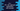# C++ STL std::replace() function explanation with example## C++ STL std::replace() function explanation with example:

replace is defined in algorithm header in C++. We can use this method to replace one value with a new value in a given range. In this post, we will learn how to use replace with example.

### Syntax of std::replace():

Below is the syntax of std::replace():

``void replace(Iterator first, Iterator last, const T& old, const T& new)``

Here, first : It is the forward iterator to the initial position of the sequence last : It is the forward iterator the the last position of the sequence old : Old value to be replaced new : New value to replace with old

This method replaces the old value with new in range first and last.

### Return value of std::replace():

It’s return value is void or it don’t return any value. It uses operator == to compare the new value with old value.

### C++ program:

Below is the complete C++ program :

``````#include<algorithm>
#include<iostream>

using namespace std;

void printArray(int *arr, int size){
for(int i = 0; i< size; i++)
cout<<arr[i]<<" ";

cout<<endl;
}

int main(){
int givenArr[] = {-1, -1, -1, -1, -1, -1, -1, -1, -1, -1, -1, -1, -1, -1};
int size = sizeof(givenArr)/sizeof(givenArr);

cout<<"Given Array :"<<endl;
printArray(givenArr, size);

replace(givenArr, givenArr + 10, -1, 0);

cout<<"Modified Array :"<<endl;
printArray(givenArr, size);

return 0;
}``````

Here,

• We are using header. But we can also use <bits/stdc++.h> .
• printArray is a function to print the contents of an array. We can pass one array and its length to this function and it will print the array.
• givenArr is the given array. This array is filled with -1 at the beginning of the program.
• size is the size of givenArr.
• We are printing the content of the array before and after using replace. We are using replace to replace all contents in the array from starting index 0 to index 9. It replaces -1 with 0.

### Output:

If you run this program, it will print the below output:

``````Given Array :
-1 -1 -1 -1 -1 -1 -1 -1 -1 -1 -1 -1 -1 -1
Modified Array :
0 0 0 0 0 0 0 0 0 0 -1 -1 -1 -1``````